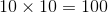# GRE Subject Test: Biology : Microscopy

## Example Questions

### Example Question #1 : Understanding Light Microscopy

To what power would a microscope magnify an object if the 10x low-power objective were in place and the microscope has a standard eyepiece?

1000x

10000x

100x

10x

1x

100x

Explanation:

The occular, or eye piece, has a magnification power of 10x. If the 10x low-power objective were in place, the magnification would be multiplicative.### Example Question #1 : Understanding Light Microscopy

Which term is used to describe the smallest structure that can be seen by a light microscope?

Visual acuity

Resolving power

Optical transmission

Index of refraction

Optotype

Resolving power

Explanation:

Optical transmission is the amount of light that passes through a laboratory specimen. It can be used to measure the concentration of components in the specimen. Optotype is the variable-sized type used in testing visual acuity. Visual acuity is a measure of the resolving power of the eye; the smallest type that can be seen at a distance. The index of refraction is defined as the speed of light in a vacuum divided by the speed of light in a medium.

The resolving power of a light microscope is the smallest structure that can be seen, approximately 1 micrometer (a millionth of a meter) by the microscope.

### Example Question #2 : Understanding Light Microscopy

When using a light microscope, the light waves that pass through or bounce off of a specimen are focused and magnified by which of the following?

Occulars

Lens

Iris

Diaphgram

Fine focus

Lens

Explanation:

Light microscopes use lenses that range from 4x to 100x, are made of glass, and focus and magnify light rays.

The diaphragm regulates the amount of light that passes through an object being magnified. The iris controls the diameter of the beam of light entering the lens. The fine focus is used to move the objectives (lenses) to view objects clearly. The occulars are the lenses that one would look into to view the object.

### Example Question #1 : Understanding Electron Microscopy

Which of the following is used to focus the electrons when using an electron microscope?

Magnetic field

Lens

Phase plate

Reticle

Laser

Magnetic field

Explanation:

Electron microscopes use beams of electrons instead of light. The electrons are focused by magnetic fields rather than by lenses.

A phase plate is used with a light microscope to view details of objects characterized by differences in refractive index and, as such, have a difference in brightness or color. Tissues deep within the body can be imaged using the technique of multiphoton microscopy, in which photons are directed into tissues by an infrared laser. The reticle is a tiny grid pattern inserted into the eyepiece lens. It is used to make measurements of objects seen through the lens.

### Example Question #2 : Understanding Electron Microscopy

Which of the following is not a type of the electron microscope?

Scanning transmission electron microscope

Transmission electron microscope

Retraction electron microscope

Reflection electron microscope

Scanning electron microscope